Ex 6.3

Chapter 6 Class 12 Application of Derivatives (Term 1)
Serial order wise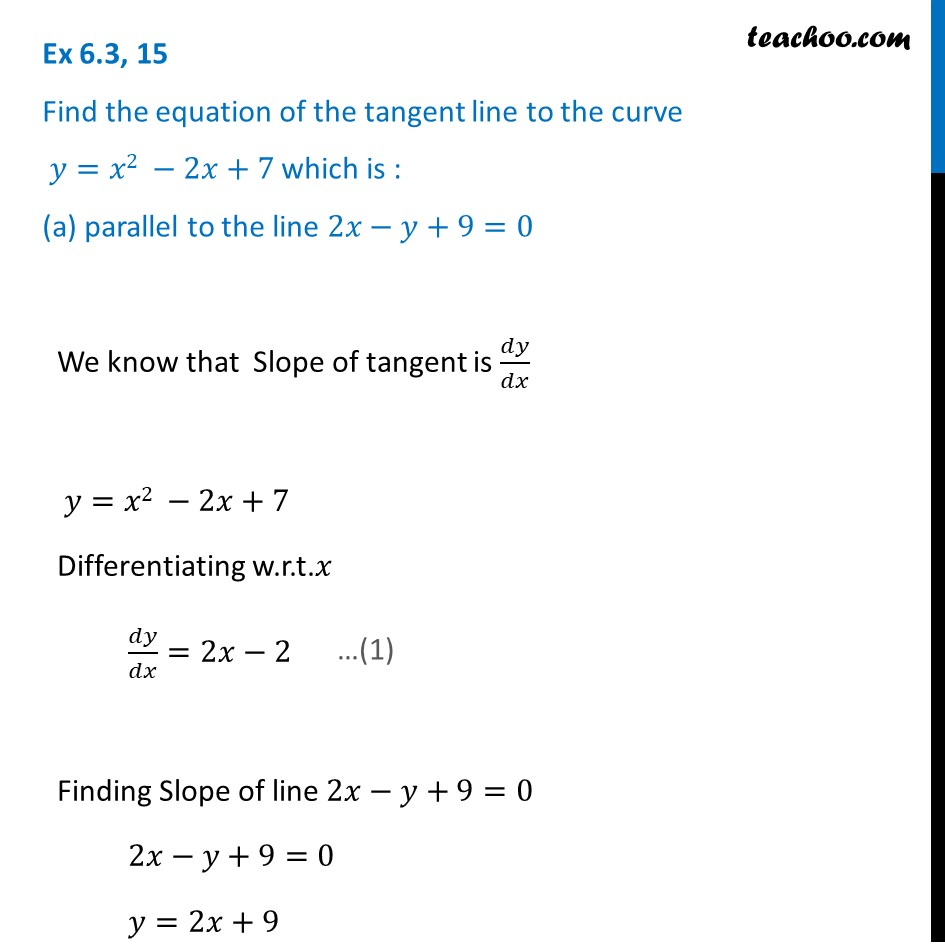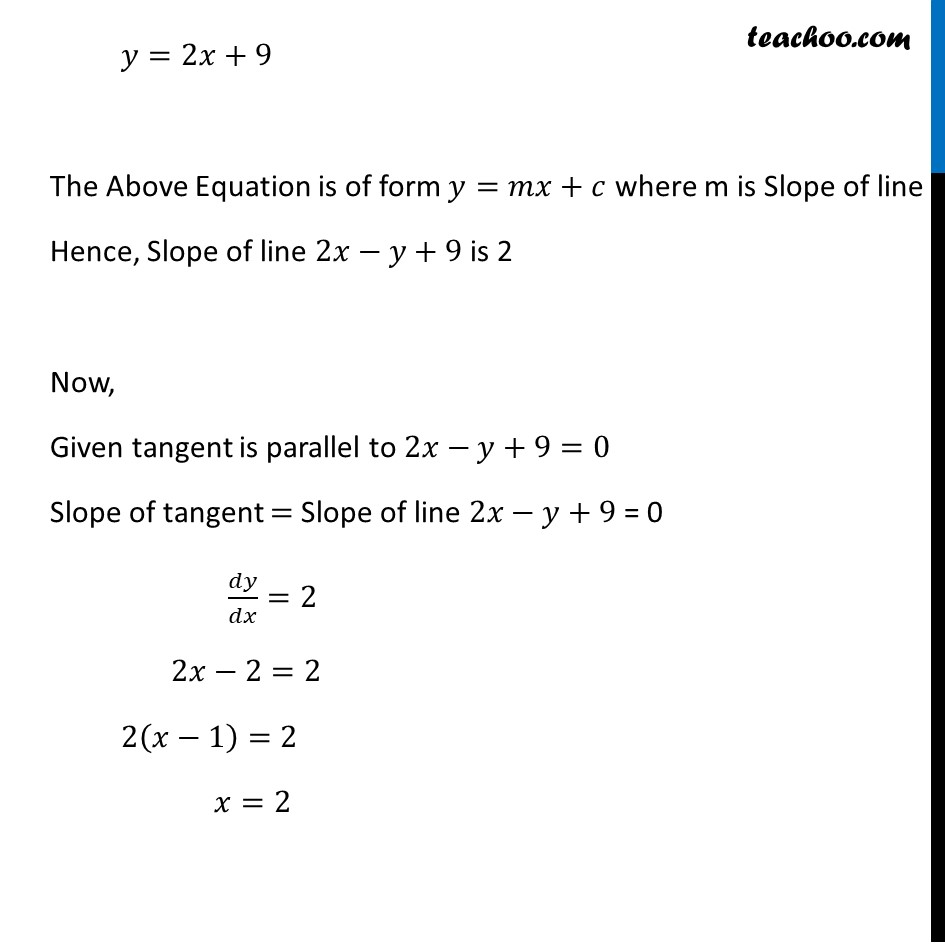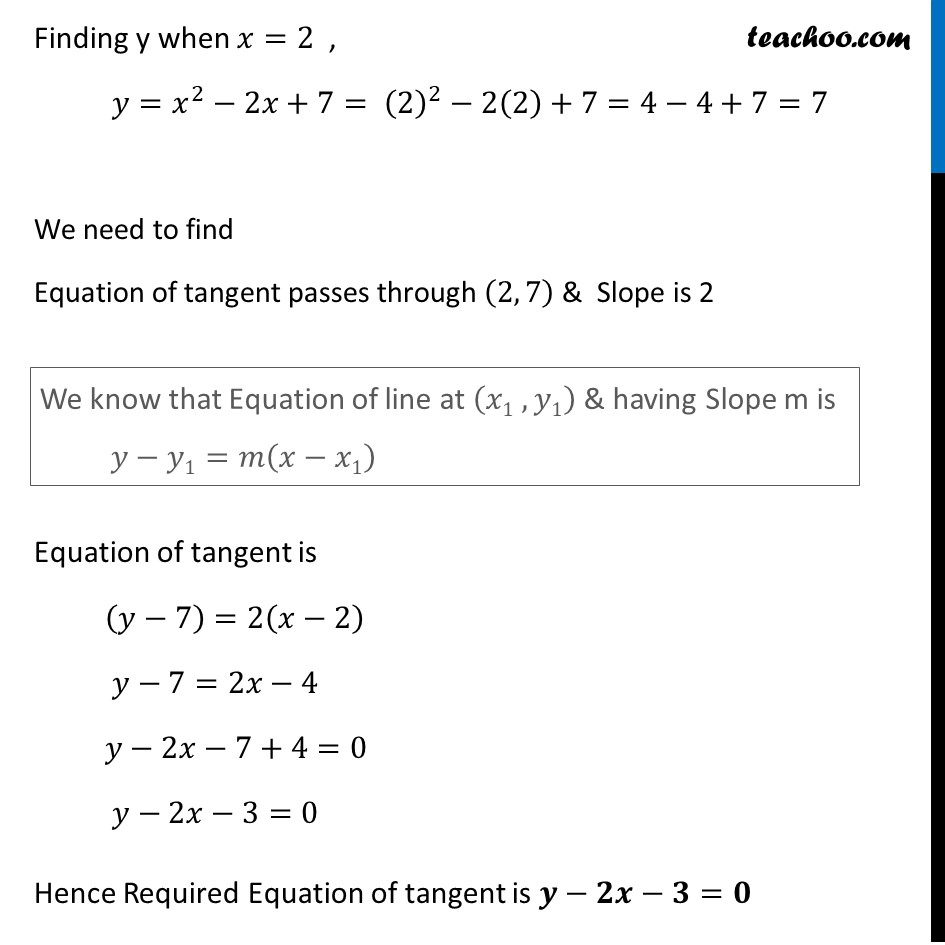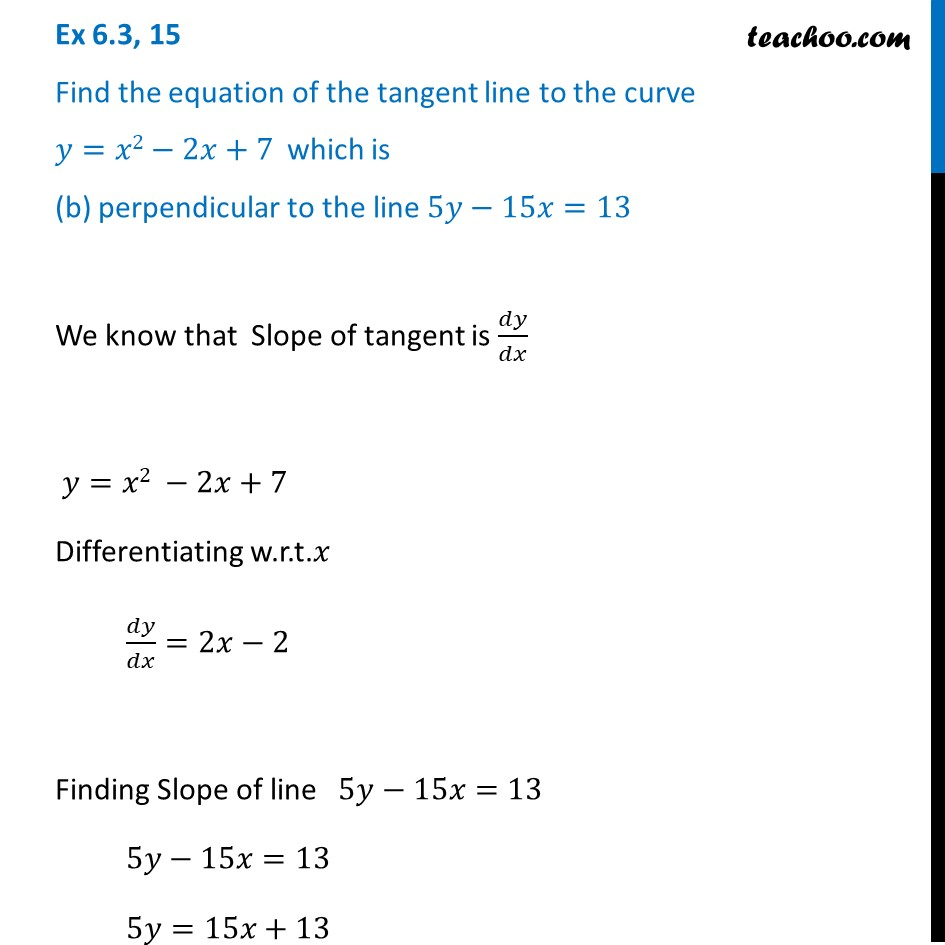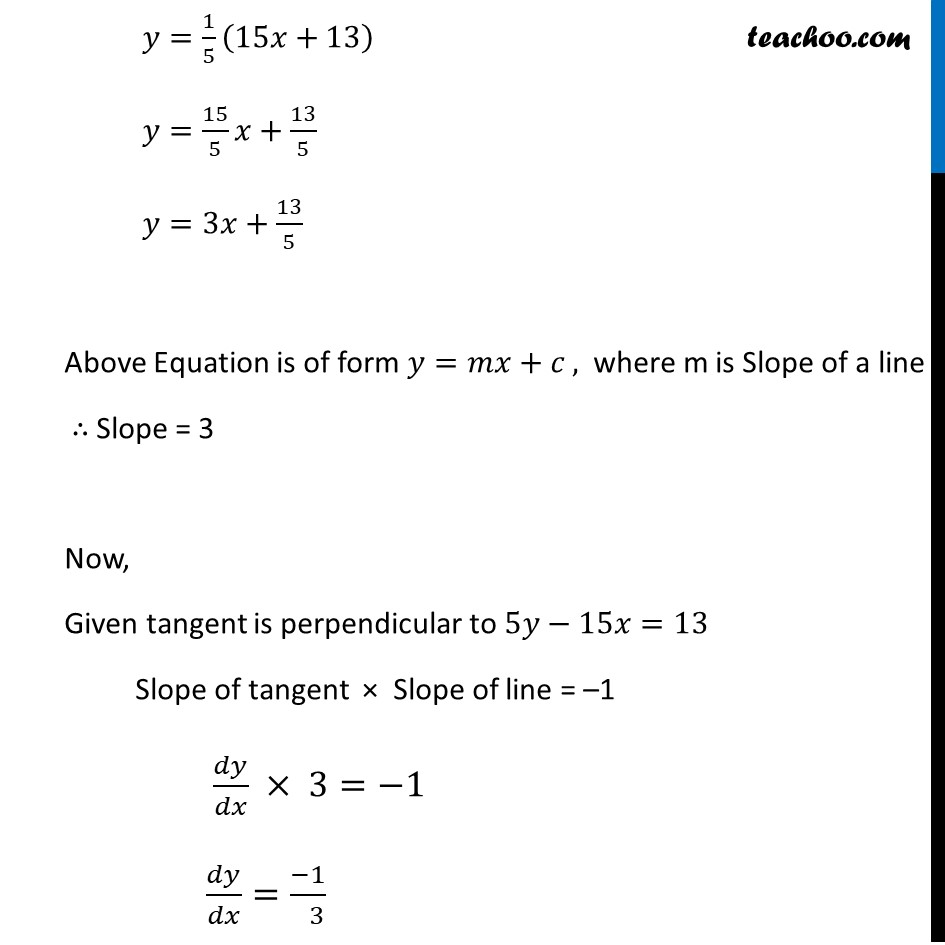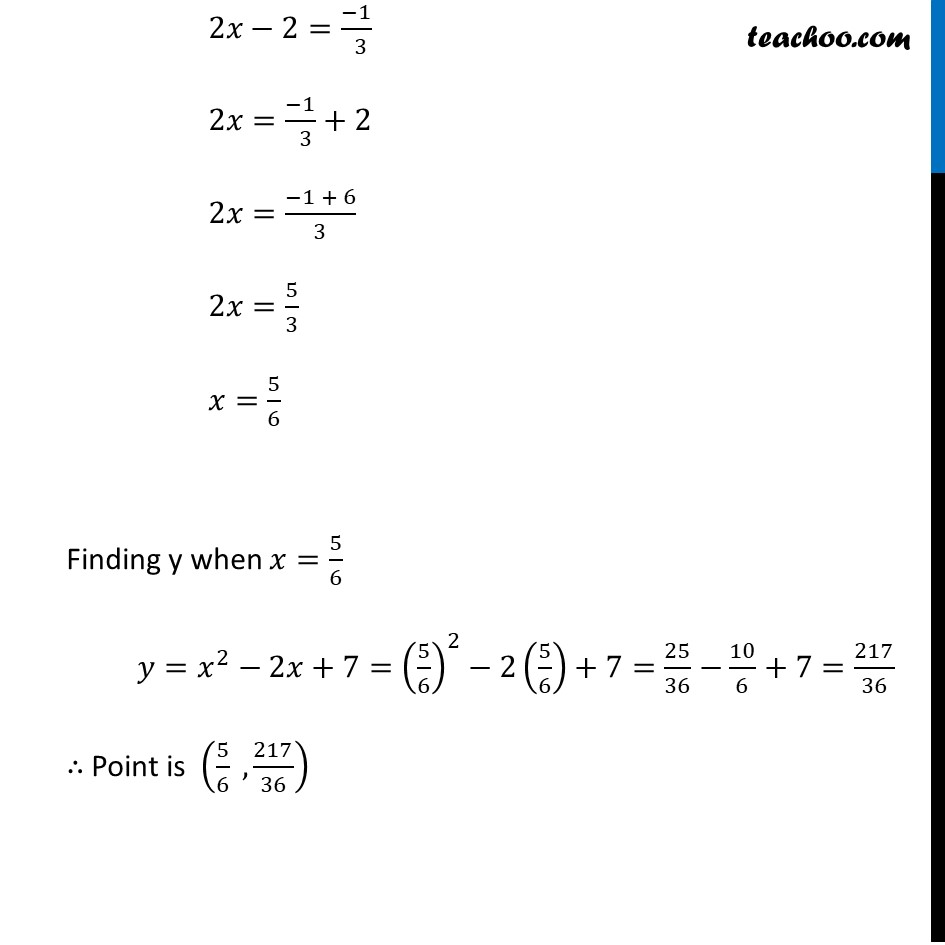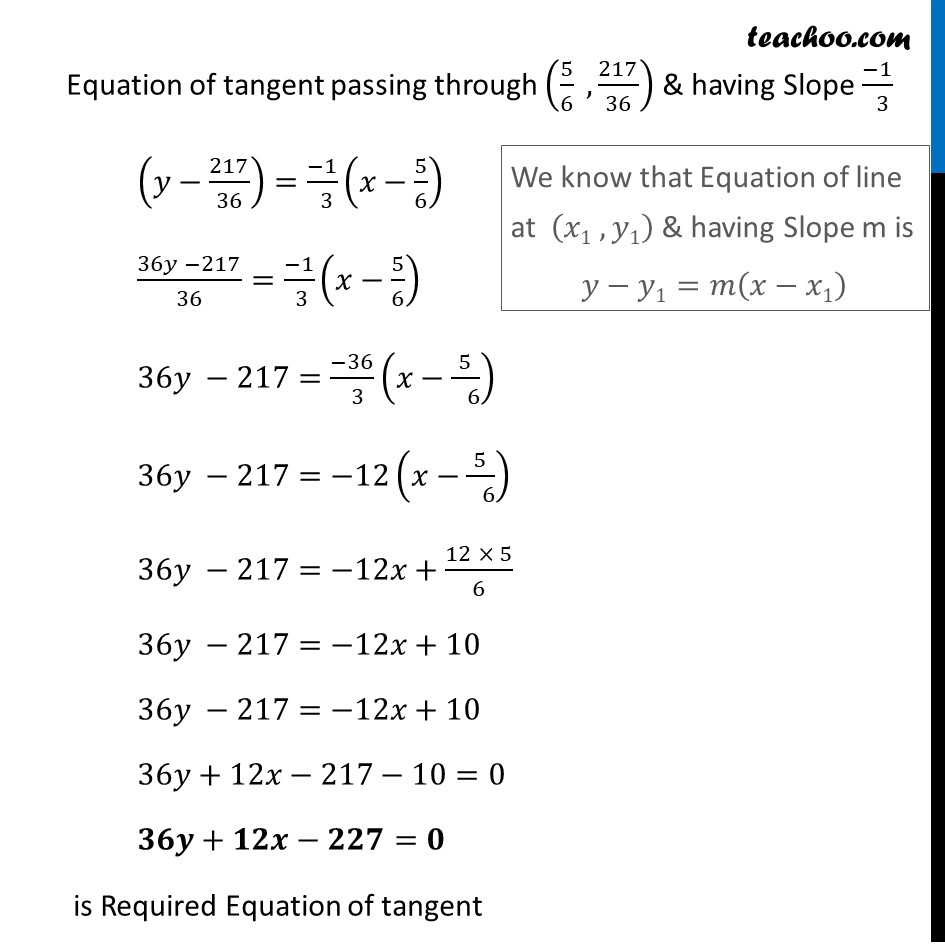### Transcript

Ex 6.3, 15 Find the equation of the tangent line to the curve 𝑦=𝑥2 −2𝑥+7 which is : (a) parallel to the line 2𝑥−𝑦+9=0We know that Slope of tangent is 𝑑𝑦/𝑑𝑥 𝑦=𝑥2 −2𝑥+7 Differentiating w.r.t.𝑥 𝑑𝑦/𝑑𝑥=2𝑥−2 Finding Slope of line 2𝑥−𝑦+9=0 2𝑥−𝑦+9=0 𝑦=2𝑥+9 𝑦=2𝑥+9 The Above Equation is of form 𝑦=𝑚𝑥+𝑐 where m is Slope of line Hence, Slope of line 2𝑥−𝑦+9 is 2 Now, Given tangent is parallel to 2𝑥−𝑦+9=0 Slope of tangent = Slope of line 2𝑥−𝑦+9 = 0 𝑑𝑦/𝑑𝑥=2 2𝑥−2=2 2(𝑥−1)=2 𝑥=2 Finding y when 𝑥=2 , 𝑦=𝑥^2−2𝑥+7= (2)^2−2(2)+7=4−4+7=7 We need to find Equation of tangent passes through (2, 7) & Slope is 2 Equation of tangent is (𝑦−7)=2(𝑥−2) 𝑦−7=2𝑥−4 𝑦−2𝑥−7+4=0 𝑦−2𝑥−3=0 Hence Required Equation of tangent is 𝒚−𝟐𝒙−𝟑=𝟎 We know that Equation of line at (𝑥1 , 𝑦1) & having Slope m is 𝑦−𝑦1=𝑚(𝑥−𝑥1) Ex 6.3, 15 Find the equation of the tangent line to the curve 𝑦=𝑥2−2𝑥+7 which is (b) perpendicular to the line 5𝑦−15𝑥=13We know that Slope of tangent is 𝑑𝑦/𝑑𝑥 𝑦=𝑥2 −2𝑥+7 Differentiating w.r.t.𝑥 𝑑𝑦/𝑑𝑥=2𝑥−2 Finding Slope of line 5𝑦−15𝑥=13 5𝑦−15𝑥=13 5𝑦=15𝑥+13 𝑦=1/5 (15𝑥+13) 𝑦=15/5 𝑥+13/5 𝑦=3𝑥+13/5 Above Equation is of form 𝑦=𝑚𝑥+𝑐 , where m is Slope of a line ∴ Slope = 3 Now, Given tangent is perpendicular to 5𝑦−15𝑥=13 Slope of tangent × Slope of line = –1 𝑑𝑦/𝑑𝑥 × 3=−1 𝑑𝑦/𝑑𝑥=(−1)/( 3) 2𝑥−2=(−1)/( 3) 2𝑥=(−1)/( 3)+2 2𝑥=(−1 + 6)/3 2𝑥=5/3 𝑥=5/6 Finding y when 𝑥=5/6 𝑦=𝑥^2−2𝑥+7=(5/6)^2−2(5/6)+7=25/36−10/6+7=217/36 ∴ Point is (5/6 ,217/36) Equation of tangent passing through (5/6 ,217/36) & having Slope (−1)/( 3) (𝑦−217/36)=(−1)/( 3) (𝑥−5/6) (36𝑦 −217)/36=(−1)/( 3) (𝑥−5/6) 36𝑦 −217=(−36)/( 3) (𝑥−5/( 6)) 36𝑦 −217=−12(𝑥−5/( 6)) 36𝑦 −217=−12𝑥+(12 × 5)/6 36𝑦 −217=−12𝑥+10 36𝑦 −217=−12𝑥+10 36𝑦+12𝑥−217−10=0 𝟑𝟔𝒚+𝟏𝟐𝒙−𝟐𝟐𝟕=𝟎 is Required Equation of tangent We know that Equation of line at (𝑥1 , 𝑦1) & having Slope m is 𝑦−𝑦1=𝑚(𝑥−𝑥1)